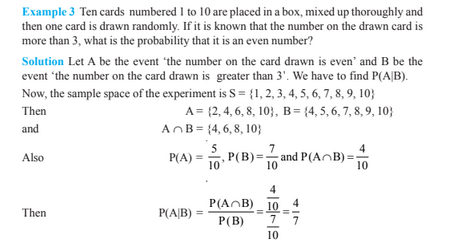# Probability For Class 12

Probability For Class 12 covers topics like conditional probability, multiplication rule, random variables, Bayes theorem, etc. Probability is defined as the extent to which an event is likely to occur. It is measured as a number of favourable events to occur from the total number of event that occurs. It is to be noted that the probability of an event is always 0 ≤ Pe ≤ 1. For example, when we toss a coin, either we get Head OR Tail, only two possible outcomes are possible (H, T). But if we toss two coins in the air, there could be three possibilities of events to occur, such as both the coins show heads or both shows tails or one shows heads and one tail, i.e.(H,H), (H,T),(T,T).

## Probability Formula

The probability formula is defined as the likelihood of an event to happen is equal to the ratio of the number of results and the total number of outcomes. The formula for the probability of an event to occur is given by;

 P(E) = Number of favourable outcomes/Total Number of outcomes

## Conditional Probability

Conditional Probability is the likelihood of an event or outcome occurring based on the occurrence of a previous event or outcome. It simply depends on any event in the past which has already taken place.

If E and F are two events with the same sample space of a random experiment, then the conditional probability of the event E gives that F has occurred, i.e. P(E|F) is,

P(E|F) = P(E ∩ F)/P(F), provided P(F) ≠ 0

### Properties of Conditional Probability

Let E and F be events of a sample space S of an experiment, then;

Property 1: P(S|F) = P(F|F) = 1

Property 2: If A and B are two events in a sample space S and F is an event of S, such that P(F)≠0, then;

P((A ∪ B)|F) = P(A|F) + P(B|F) – P((A ∩ B)|F)

Property 3: P(E′|F) = 1 − P(E|F)

### Multiplication Rule

Let E and F be two events associated with a sample space S. Clearly, the set E ∩ F denotes the event that both E and F have occurred. In other words, E ∩ F denotes the simultaneous occurrence of the events E and F. The event E ∩ F is also written as EF. According to this rule, if E and F are the events in a sample space, then;

P(E ∩ F) = P(E) P(F|E) = P(F) P(E|F)

where P(E) ≠ 0 and P(F) ≠ 0

### Independent Events

Two experiments are said to be independent if for every pair of events E and F, where E is associated with the first experiment and F with the second experiment, the probability of the simultaneous occurrence of the events E and F when the two experiments are performed is the product of P(E) and P(F) calculated separately on the basis of two experiments, i.e.,

P (E ∩ F) = P (E) . P(F)

### Probability Examples